# “20-20” Quantitative Aptitude | Crack SBI Clerk 2018 Day-24

Dear Friends, SBI Clerk 2018 Notification has been released we hope you all have started your preparation. Here we have started New Series of Practice Materials specially for SBI Clerk 2018. Aspirants those who are preparing for the exams can use this “20-20” Quantitative Aptitude Questions.

## Daily Practice Test Schedule | Good Luck

 Topic Daily Publishing Time Daily News Papers & Editorials 8.00 AM Current Affairs Quiz 9.00 AM Logical Reasoning 10.00 AM Quantitative Aptitude “20-20” 11.00 AM Vocabulary (Based on The Hindu) 12.00 PM Static GK Quiz 1.00 PM English Language “20-20” 2.00 PM Banking Awareness Quiz 3.00 PM Reasoning Puzzles & Seating 4.00 PM Daily Current Affairs Updates 5.00 PM Data Interpretation / Application Sums (Topic Wise) 6.00 PM Reasoning Ability “20-20” 7.00 PM English Language (New Pattern Questions) 8.00 PM General / Financial Awareness Quiz 9.00 PM
[WpProQuiz 1293]

Click “Start Quiz” to attend these Questions and view Explanation

### Click Here for SBI Clerk Prelims and Mains 2018 – Full Length Mock Test

Directions (Q. 1-5): What should come in place of question mark (?) in the following questions?

1). 12.8% of 8800 – 16.4% of 5550 = 10% of ?

1. 964
2. 2162
3. 2062
4. 1982
5. 2226

2). (2197)2/3 ÷  (28561)3/4 × ? = (√13)5

1. (13)2
2. (√13)-2
3. (13)7
4. (√13)7
5. (√13)-7

3). [(6√11+√11) ×(7√11+9√11)] – (28)2= ?

1. 454
2. 484
3. 462
4. 478
5. 448

4). 8 (9/47) × 9 (13/56) × 7 (7/11) ÷ 2 (4/9) – 6 (3/5) = ?

1. 488.4
2. 420
3. 223.6
4. 413.6
5. 229.65

5).1739 ÷ 47 + 2679 ÷ 57 + 3819 ÷ 67 +5159 ÷ 77 + 6699 ÷ 87 + 1245 ÷ 83 = ?

1. 315
2. 300
3. 288
4. 295
5. 324

Directions (Q. 6-10): In each, these questions a number series is given. In each series only one number is missing. Find out the number.

6). 97, 108, 130, 163,  ? ,   262

1. 230
2. 210
3. 217
4. 207
5. 202

7). 15,  28,  45,  66 , ? ,  120

1. 105
2. 86
3. 98
4. 91
5. 84

8). 65,  83,  105,  131,  161, ?

1. 140
2. 165
3. 195
4. 194
5. 160

9). 11,  25,  55,  117,   ?,   497

1. 243
2. 235
3. 248
4. 234
5. 245

10). 1,  4,   19,  54,  117,   ?

1. 195
2. 216
3. 215
4. 205
5. 208

Directions (Q. 11-15): Study the graphs carefully and answer the questions that follow:

The bar graph shows the number of candidates appeared in IBPS Clerk examination from six different states and another bar graph shows the ratio of candidates passed and failed in the states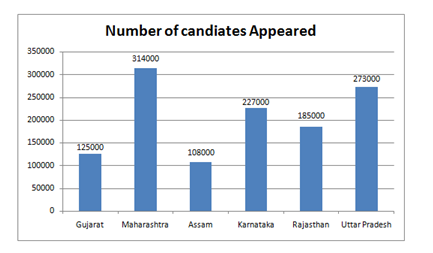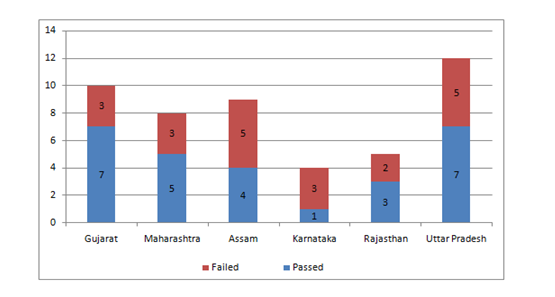1. What is the respective ratio of the number of candidates failed in the Exam from Karnataka and Rajasthan together to those failed in the exam from Gujarat and Maharashtra together?
1. 927 : 623
2. 621 : 977
3. 977 : 621
4. 947 : 459
5. 927 : 611
1. The number of candidates passed from Rajasthan and Assam together is what percent of the total number of failed candidates from Karnataka?
1. 95.78%
2. 91.28%
3. 95.49%
4. 92.01%
5. 93.39%
1. The number of candidates appearing for the exam from Assam and Gujarat together is what percent of the total number of candidates appearing for the exam from Maharashtra and Uttar Pradesh together? (rounded off to the nearest integer)
1. 45
2. 40
3. 35
4. 33
5. 30
1. Number of candidates passing in the exam from Uttar Pradesh is what percent of the total number of candidates appearing from all the States together? (rounded off to two digits after the decimal)
1. 12.93
2. 11.46
3. 13.84
4. 15.27
5. 14.52
1. What is the difference between the state has the highest number of passing students in the exam to the state has the lowest number of students failed in the exam?
1. 132250
2. 168350
3. 164450
4. 158750
5. 148950
1. If the average age of three boys are 25 year and ratio of their ages are 4: 5: 6, then what is the age of the eldest one?
1. 25
2. 35
3. 30
4. 40
5. 20
1. A bag contains 50p, 25p and 10p coins in the ratio of 5 : 3 : 2, amounting to Rs. 276. Find the number of coins of each type respectively.
1. 450, 160, 240
2. 400, 260, 140
3. 450, 230, 180
4. 400, 240, 160
5. None of these
1. If the radius and heights of the two cylinders are 4 : 7 and 3 : 8, then what is the ratio of its volume?
1. 14 : 9
2. 6 : 49
3. 9 : 14
4. 6 : 7
5. None of these
1. A, B and C divide an amount of Rs. 5000 amongst themselves in the ratio of 5:3:2 respectively. If an amount of Rs.600 is added to each of their shares, what will be the new ratio of their shares of the amount?
1. 31 : 21 : 16
2. 15 : 16 : 12
3. 17 : 18 : 19
4. 29 : 21 : 15
5. None of these

20) A shopkeeper sold an article for Rs. 1,380 at a loss of 8%. At what price should it be sold to gain a profit of 8%?

1. Rs.1,560/-
2. Rs.1,620/-
3. Rs.1,680/-
4. Rs.1,740/-
5. None of these

Directions (Q. 1-5):

Explanation:

? = [ (12.8×88 -16.4×55.5) × 100 ] / 10

= (1126.4 – 910.2) × 10 = 216.2 × 10

= 2162

Explanation:

[ (13)3]2/3 ÷ [(13)4]3/4 × ? = (√13)5

Or, 132 ÷ 133 × ? = (√13)5

Or, 13-1 × ? = (√13)5

Or, ? = 13 ×(√13)5 = (√13)7

Explanation:

? = 7√11 × 16√11 – 784

= 1232 – 784 = 448

Explanation:

? = (385/47) × (517/56) × (84/11) × (9/22) – (33/5)

= (385×27)/44 – (33/5) = (51975 – 1452) / 220

= 50523/220 = 229.65

Explanation:

? = 1739÷ 47 + 2679 ÷ 57 + 3819 ÷ 67 + 5159 ÷ 77 + 6699 ÷ 87 + 1245 ÷ 83

= 37 + 47 + 57 + 67 + 77 + 15 = 300

Directions (Q. 6-10):

Explanation:

The series is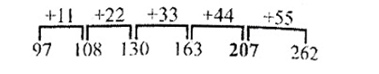Explanation:

The difference of difference are, 4,4,4,4..

Explanation:

The series is 82 + 12 + 02 = 65, 92+ 12 + 12 = 83, 102+ 12 + 22= 105, 112 + 12 + 32 = 131, 122 + 12+ 42= 161, 132+12+ 52= 195,

or, +18, +22,+26, +30, +34,

Explanation:

The series is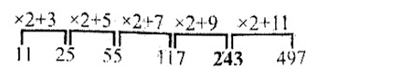Explanation:

The series is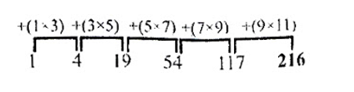Directions (Q. 11-15):

Explanation:

Number of candidates failed in the Exam from Karnataka and Rajasthan together

= 170250 + 74000 = 244250

Number of candidates failed in the exam from Gujarat and Maharashtra together

= 37500 + 117750 = 155250

Required ratio

= 244250 : 155250

= 977 : 621

Explanation:

The number of candidates passed from Rajasthan and Assam together

= 111000 + 48000 = 159000

Total number of failed candidates from Karnataka = 170250

Required percentage

= (159000/170250) × 100

= 93.39%

Explanation:

The number of candidates appearing for the exam from Assam and Gujarat together

= 108000 + 125000 = 233000

Total number of candidates appearing for the exam from Maharashtra and Uttar Pradesh together

= 314000 + 273000 = 587000

Required percentage

= 233000/587000 × 100

= 39.69

= 40%

Explanation:

Total number of candidates appearing from all the states together

= (125000 + 314000 + 108000 + 227000 + 185000 + 273000)

= 1232000

Required percentage

= 159250/1232000 × 100

= 12.93

Explanation:

State has the highest number of passing students is Maharashtra = 196250

State has the lowest number of students failed is Gujarat = 37500

Difference = 196250 – 37500

= 158750

Explanation:

The average age = 25

∴ the total age = 25 × 3 = 75

Hence, the age of the eldest boy = 6/15 × 75 = 30

Explanation:

The ratio of the 50p, 25p,10p coins be 5x,3x,2x

(5x + 3x + 2x) = 276

(5x/2 + 3x/4 + 2x/10) = 276

(50x + 15x + 4x) /20 = 276

∴ x = 80

Hence the numbers of coins are,

50p = 5 × 80 = 400

25p = 3 × 80 = 240

10p = 2 × 80 = 160

Explanation:

Volume of the cylinder = (22/7)×r^2h

Ratio of two cylinders = (22/7)× (4)^2 × 3 :(22/7)× (7)^2 × 8

= 4 × 4 × 3 : 7 × 7 × 8 = 6 : 49

Explanation:

A : B : C = 5 : 3 : 2

A = 5/10 × 5000 = 2500

B = 3/10 × 5000 = 1500

C = 2/10 × 5000 = 1000

If the amount Rs.600 is added to each of their shares then the new share becomes,

3100 : 2100 : 1600 = 31 : 21 : 16

Explanation:

Let the cost price of article = Rs. x

According to question,

x * 92/100 =1380

x = Rs.1,500/-

Required price = 1500 * 108/100

= Rs.1620

(or)

Shortcut method:

S.P1 / (100-loss%) = S.P2 /(100+Profit%)

1380/92 = S.P2 / 108

S.P2 = 1620